## Places A and B are 100 km apart on a highway.  One car starts from A and another from B at the  same time. If the cars travel in the same direction at  different speeds, they meet in 5 hours. If they travel  towards each other, they meet in 1 hour.

This question is inspired from Ex 3.5, 4 (iv) - Chapter 3 Class 10 - Pair of Linear Equations in Two Variables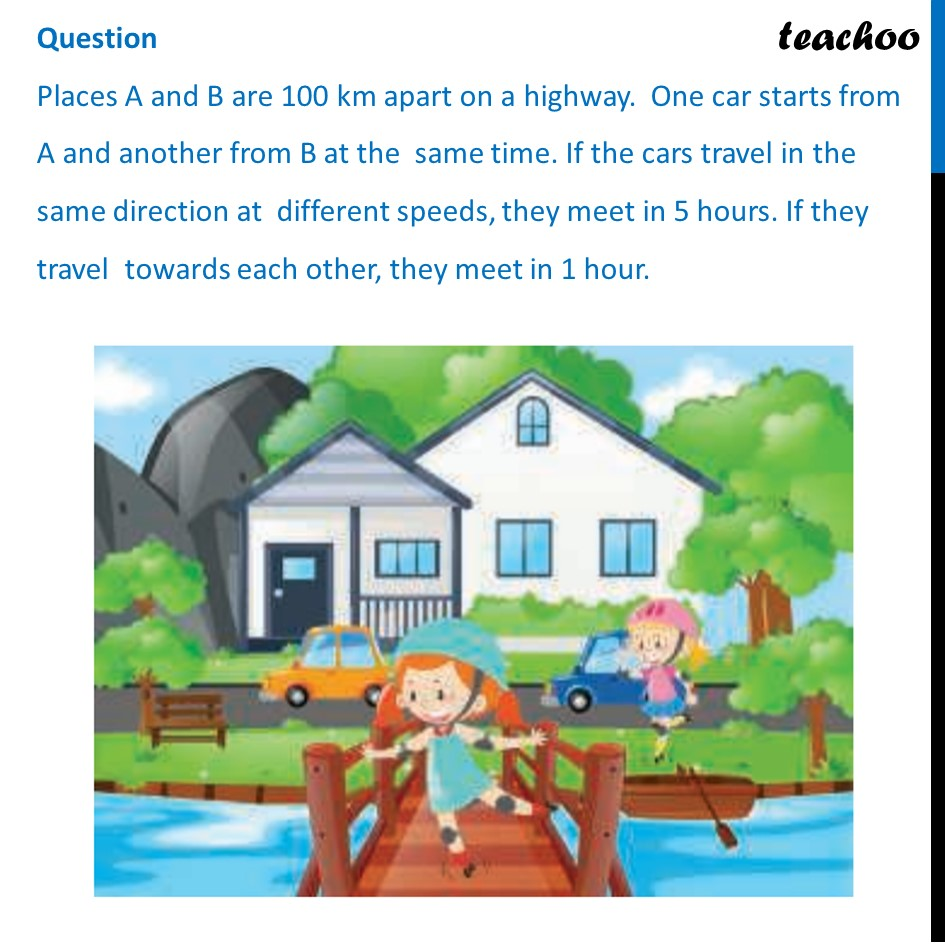## (d) ( uv ) km/hr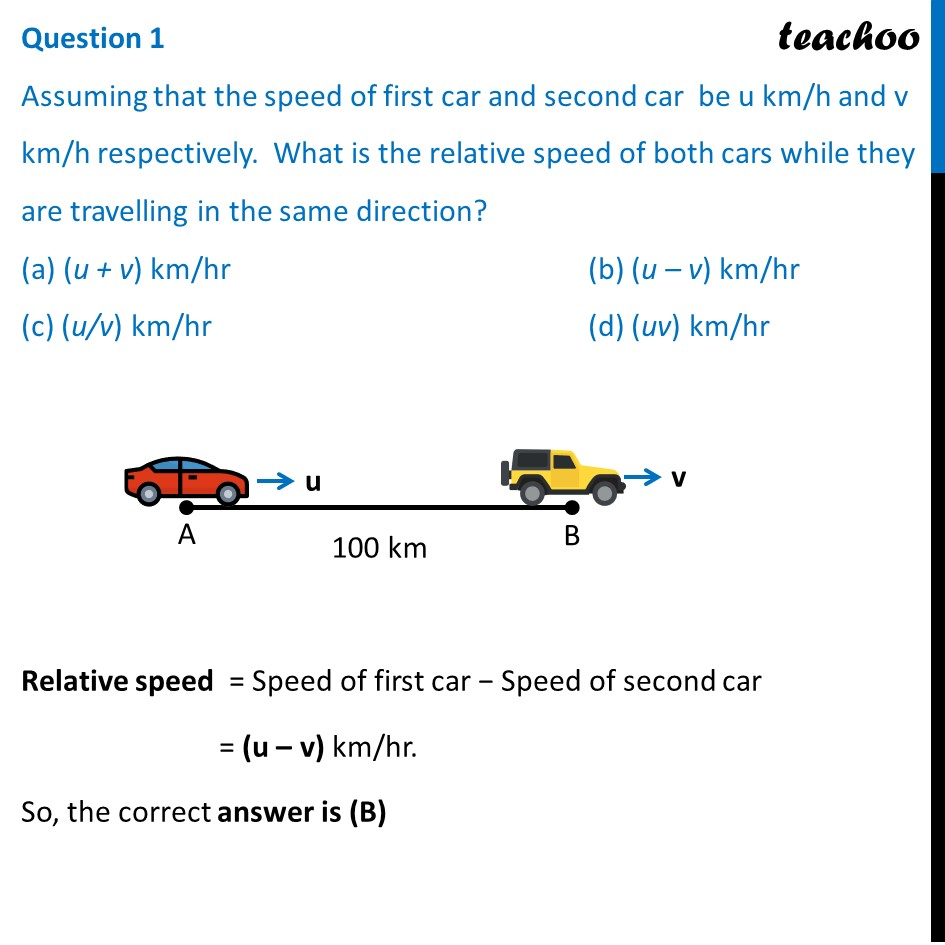## (d) ( uv ) km/hr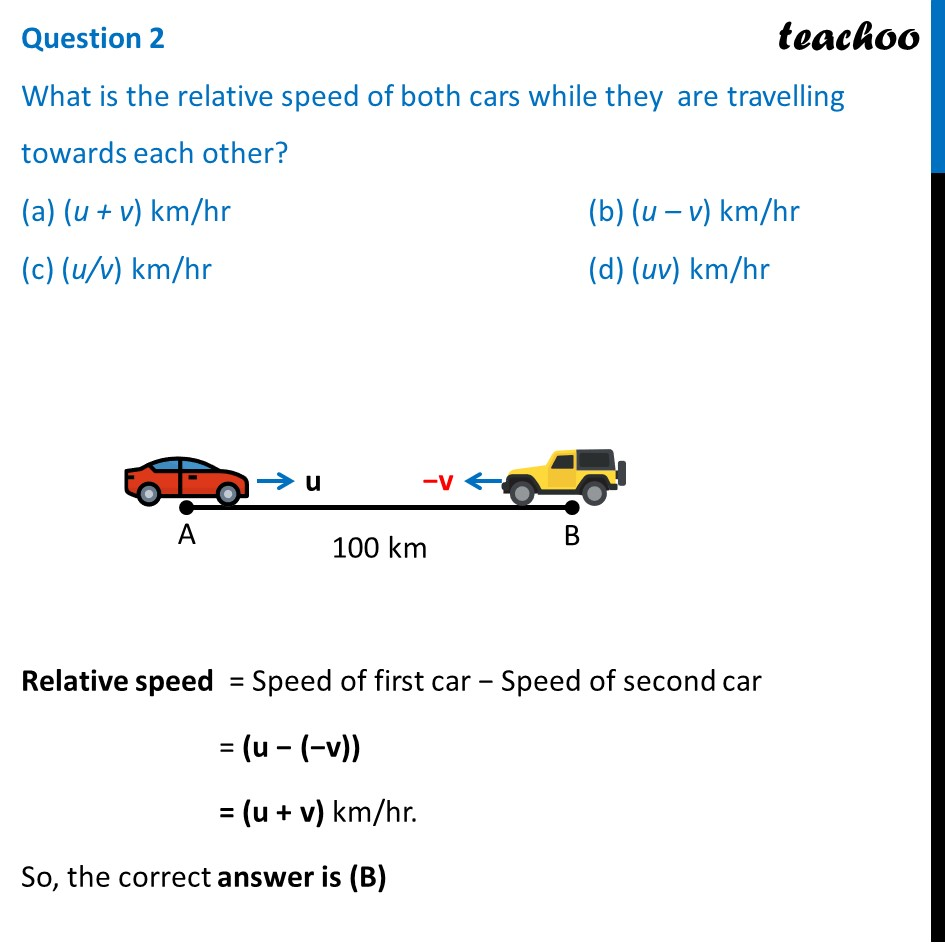## (d) 20 km/hr

This question is exactly same as Ex 3.5, 4 (iv) - Chapter 3 Class 10 , so we use the same solution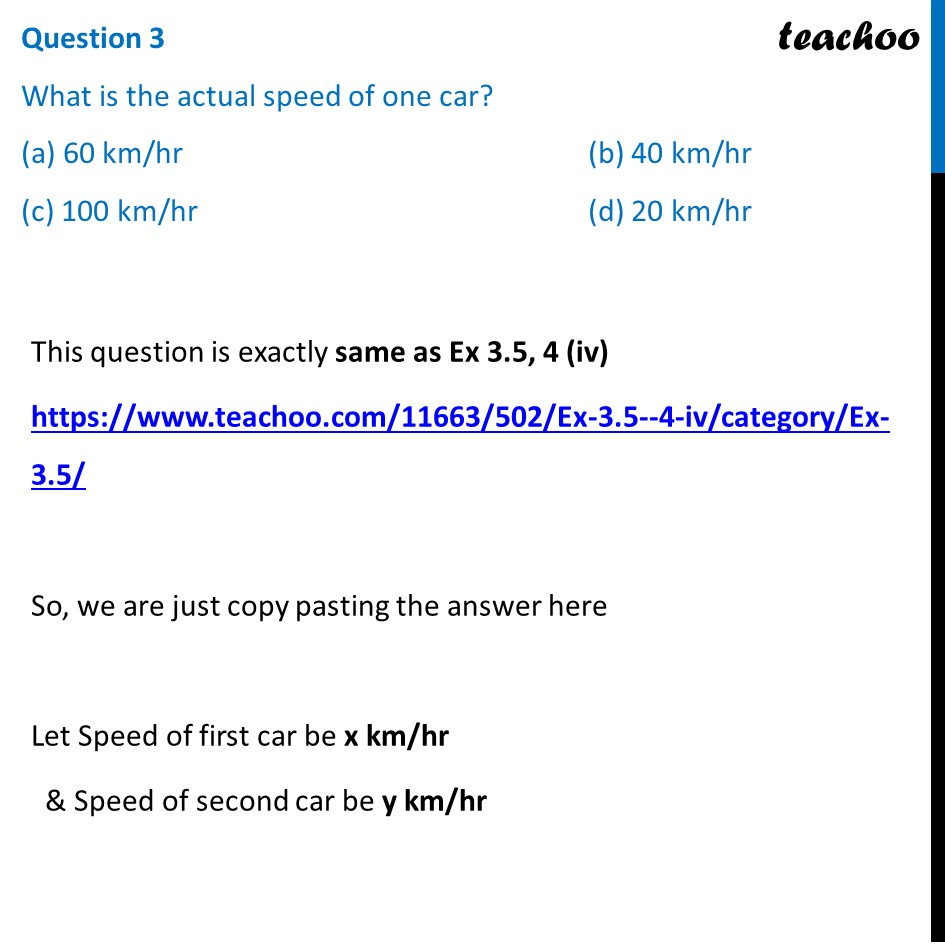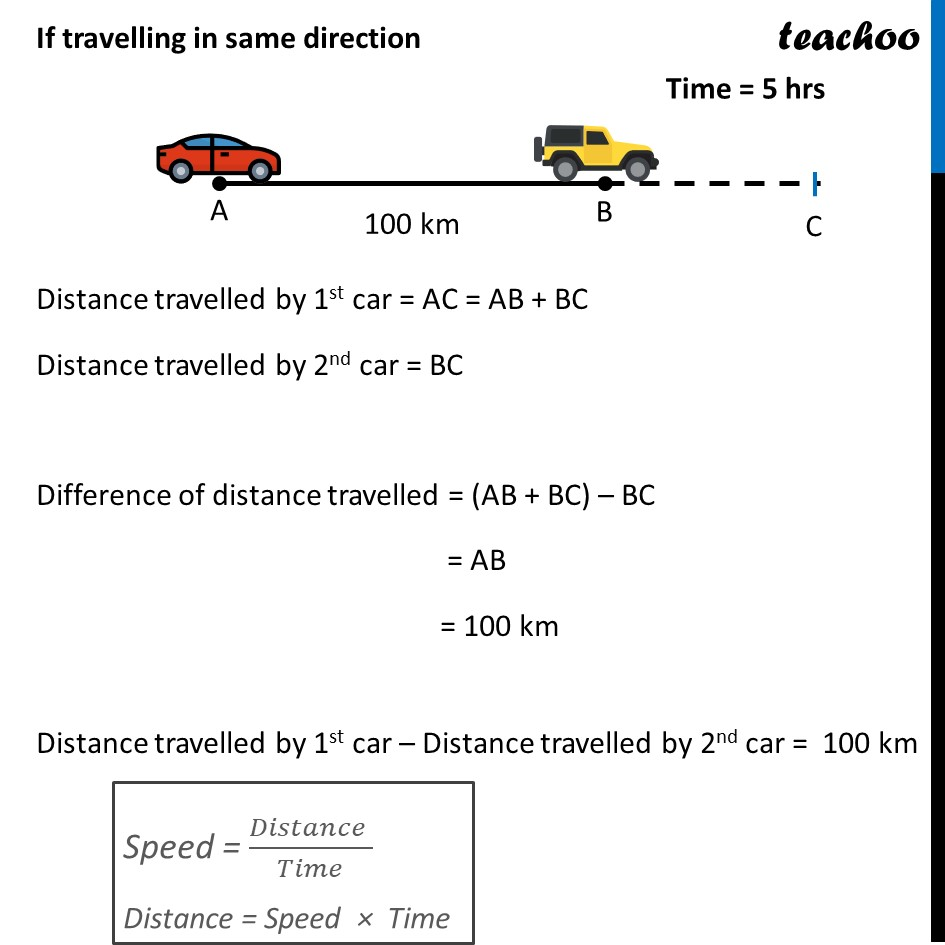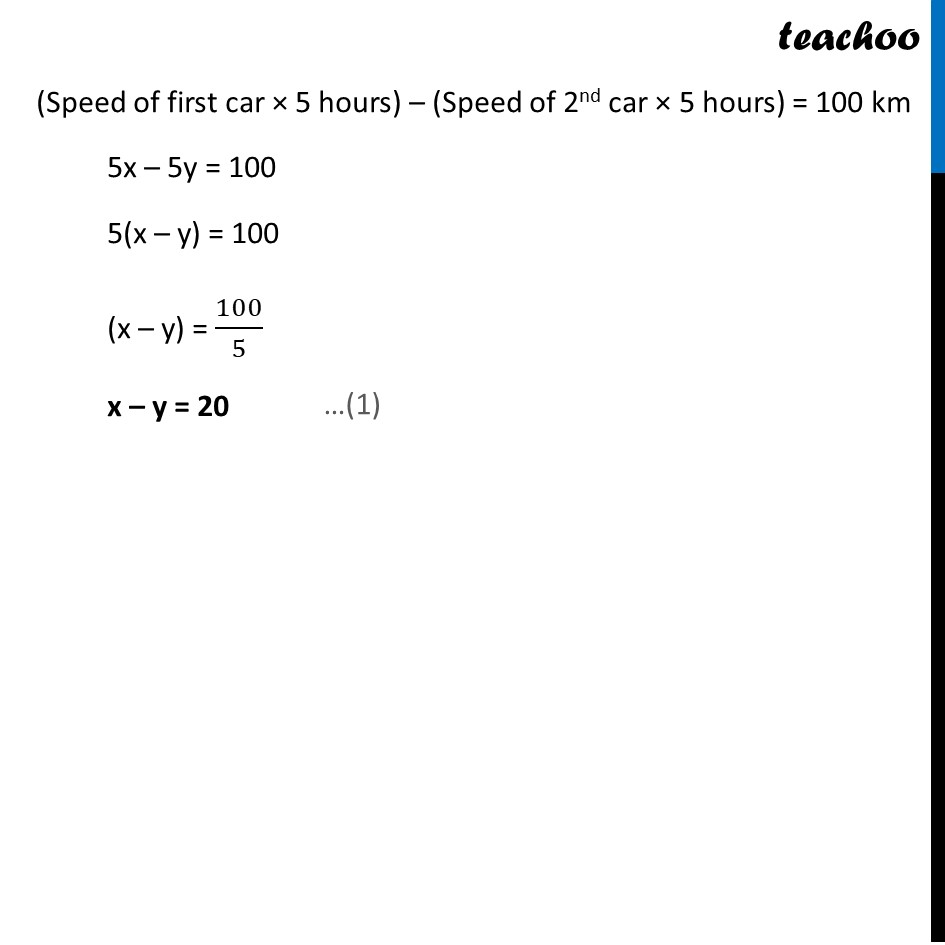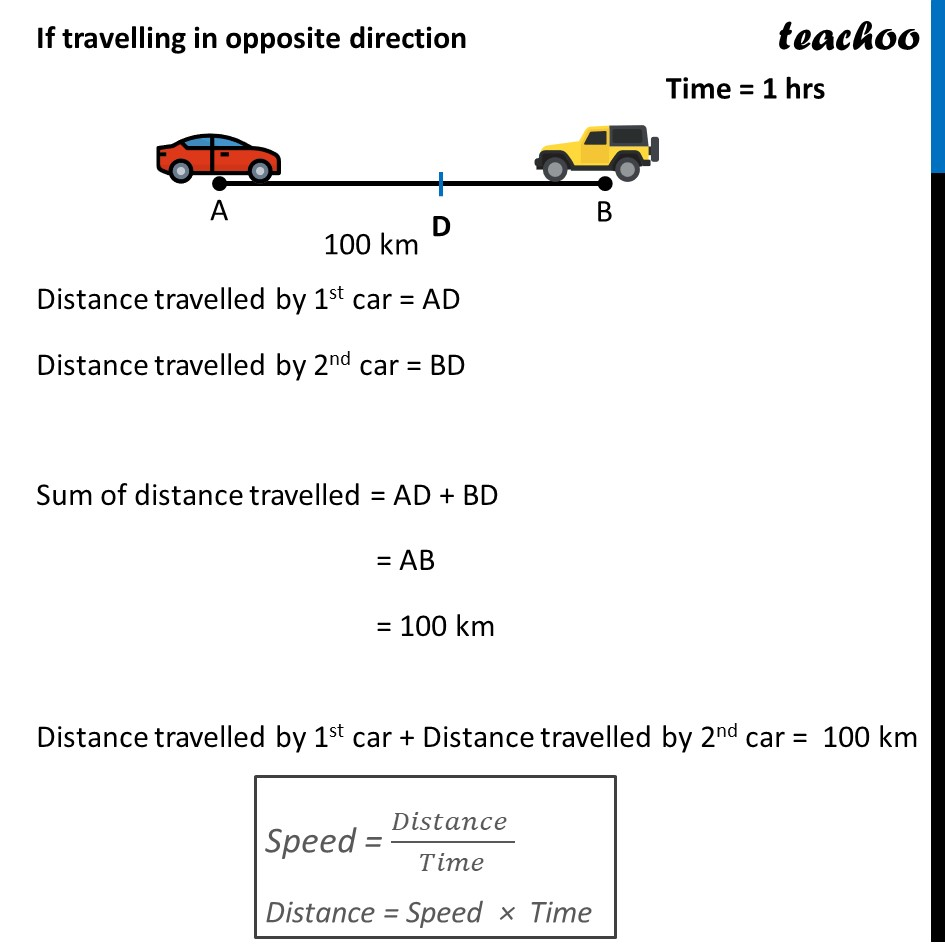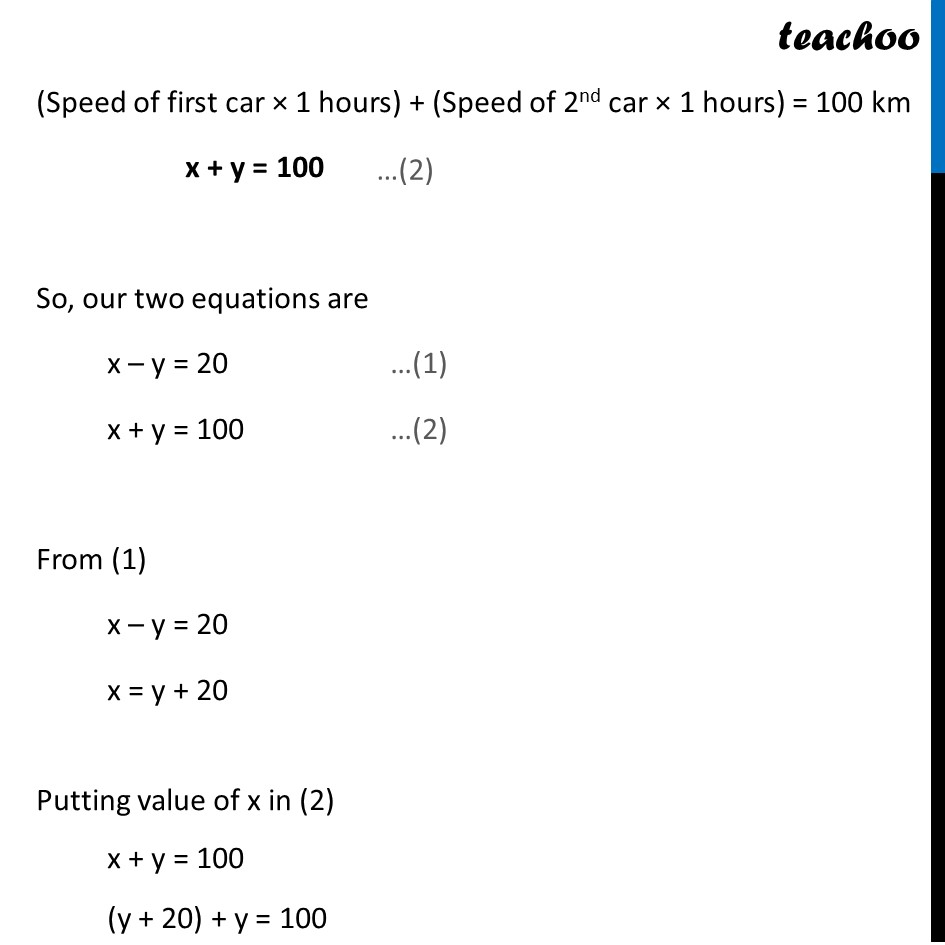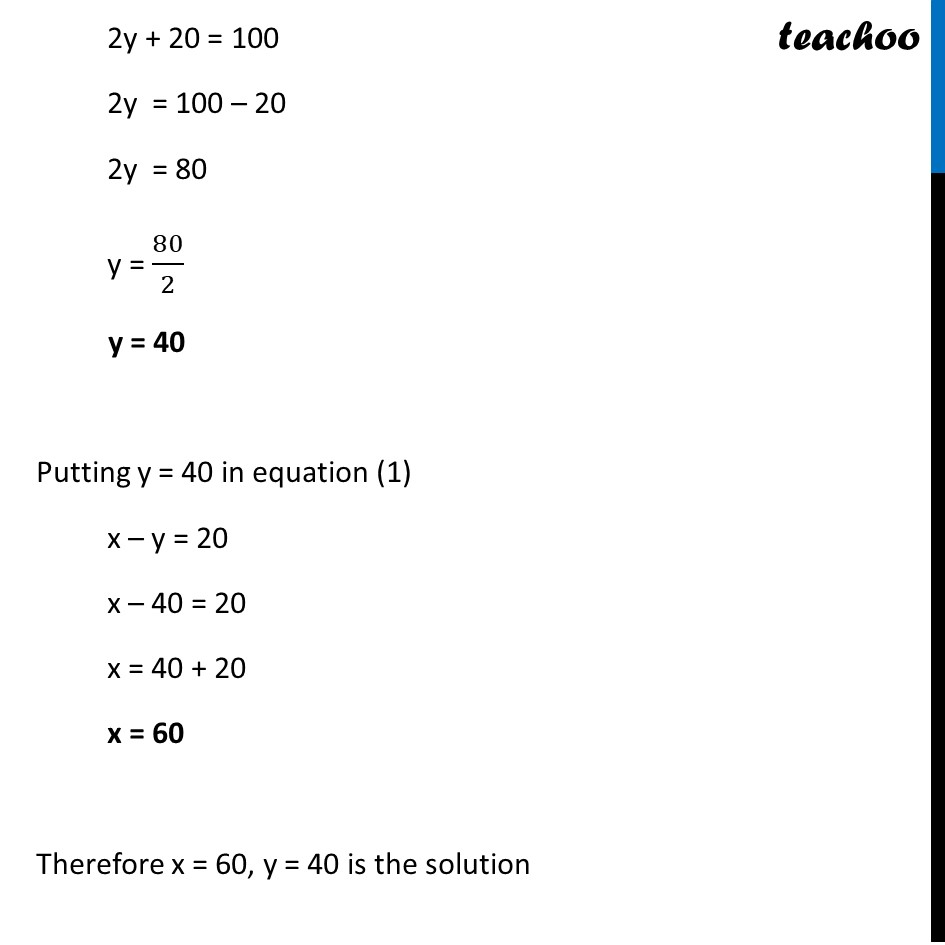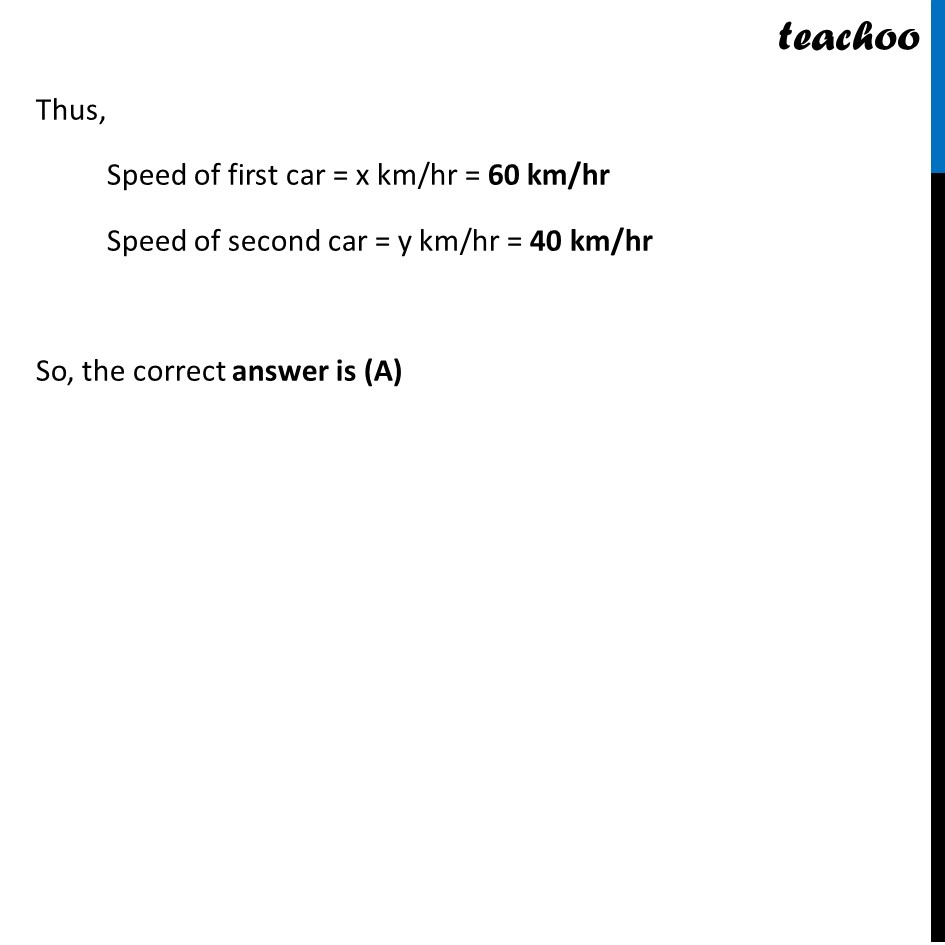## (d) 20 km/hr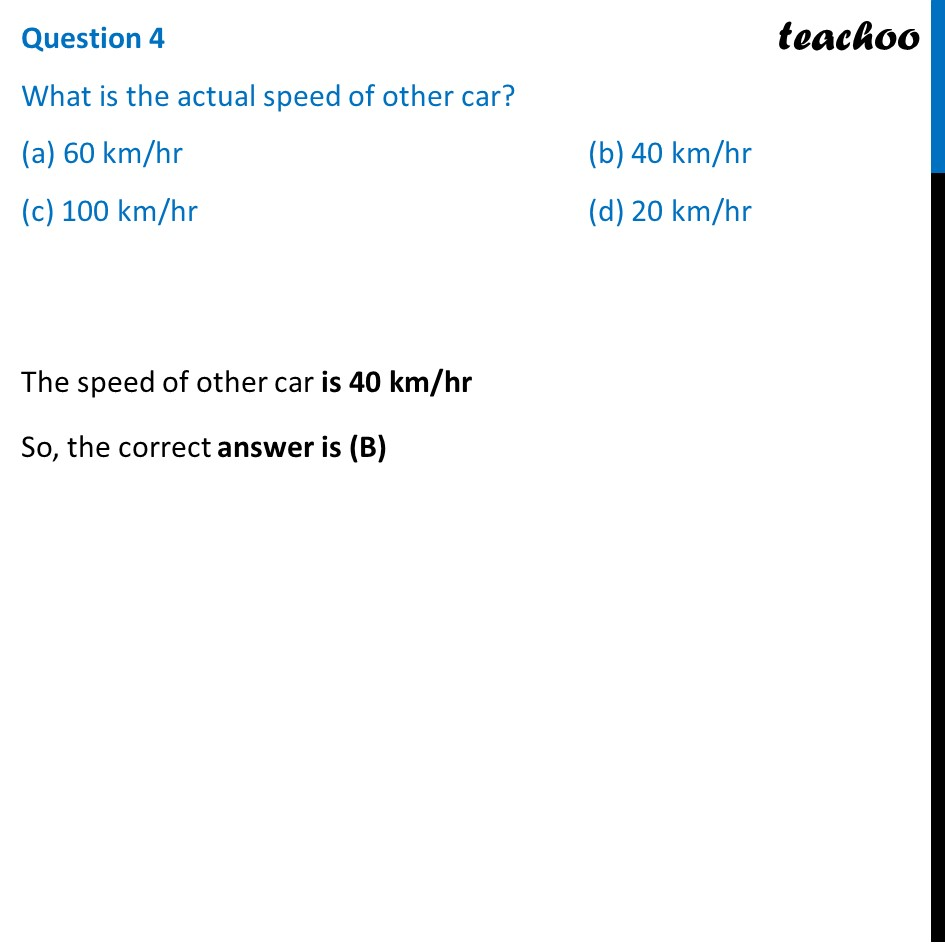## (d) none of the above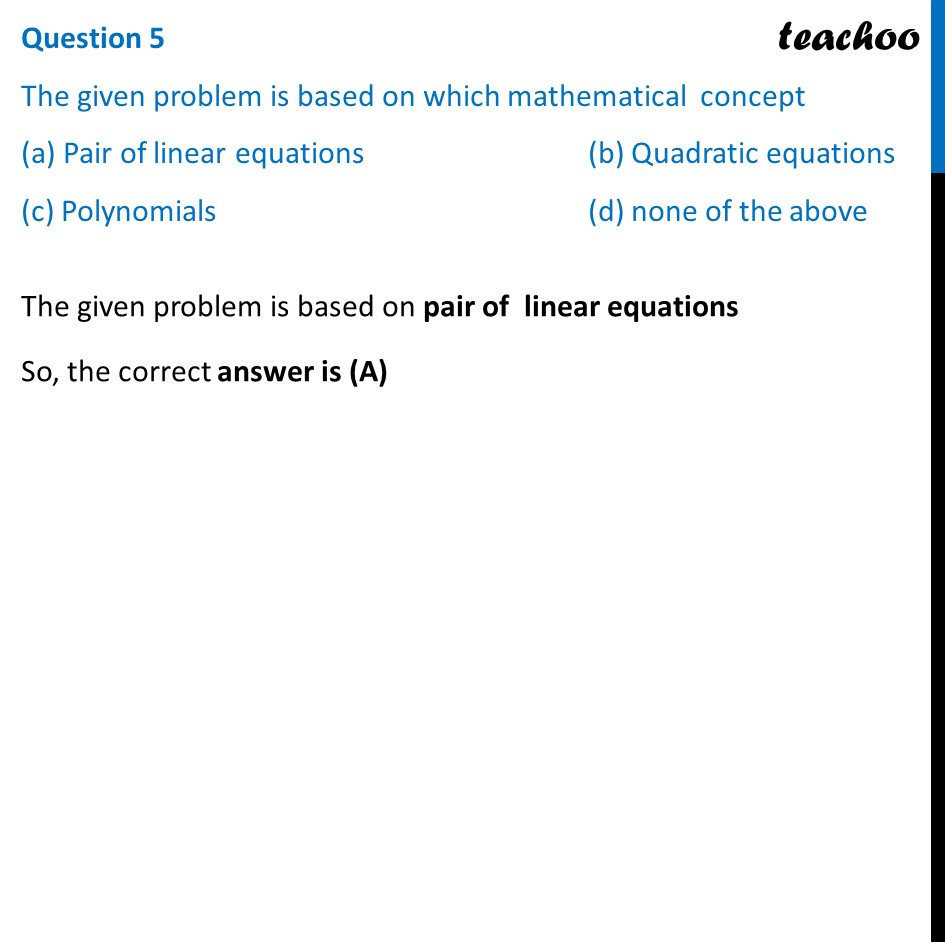1. Chapter 3 Class 10 Pair of Linear Equations in Two Variables (Term 1)
2. Serial order wise
3. Case Based Questions (MCQ)

Transcript

Question Places A and B are 100 km apart on a highway. One car starts from A and another from B at the same time. If the cars travel in the same direction at different speeds, they meet in 5 hours. If they travel towards each other, they meet in 1 hour. Question 1 Assuming that the speed of first car and second car be u km/h and v km/h respectively. What is the relative speed of both cars while they are travelling in the same direction? (a) (u + v) km/hr (b) (u – v) km/hr (c) (u/v) km/hr (d) (uv) km/hr Relative speed = Speed of first car − Speed of second car = (u – v) km/hr. So, the correct answer is (B) Question 2 What is the relative speed of both cars while they are travelling towards each other? (a) (u + v) km/hr (b) (u – v) km/hr (c) (u/v) km/hr (d) (uv) km/hr Relative speed = Speed of first car − Speed of second car = (u − (−v)) = (u + v) km/hr. So, the correct answer is (B) Question 3 What is the actual speed of one car? (a) 60 km/hr (b) 40 km/hr (c) 100 km/hr (d) 20 km/hr This question is exactly same as Ex 3.5, 4 (iv) https://www.teachoo.com/11663/502/Ex-3.5--4-iv/category/Ex-3.5/ So, we are just copy pasting the answer here Let Speed of first car be x km/hr & Speed of second car be y km/hr If travelling in same direction Distance travelled by 1st car = AC = AB + BC Distance travelled by 2nd car = BC Difference of distance travelled = (AB + BC) – BC = AB = 100 km Distance travelled by 1st car – Distance travelled by 2nd car = 100 km (Speed of first car × 5 hours) – (Speed of 2nd car × 5 hours) = 100 km 5x – 5y = 100 5(x – y) = 100 (x – y) = 100/5 x – y = 20 If travelling in opposite direction Distance travelled by 1st car = AD Distance travelled by 2nd car = BD Sum of distance travelled = AD + BD = AB = 100 km Distance travelled by 1st car + Distance travelled by 2nd car = 100 km (Speed of first car × 1 hours) + (Speed of 2nd car × 1 hours) = 100 km x + y = 100 So, our two equations are x – y = 20 …(1) x + y = 100 …(2) From (1) x – y = 20 x = y + 20 Putting value of x in (2) x + y = 100 (y + 20) + y = 100 2y + 20 = 100 2y = 100 – 20 2y = 80 y = 80/2 y = 40 Putting y = 40 in equation (1) x – y = 20 x – 40 = 20 x = 40 + 20 x = 60 Therefore x = 60, y = 40 is the solution Thus, Speed of first car = x km/hr = 60 km/hr Speed of second car = y km/hr = 40 km/hr So, the correct answer is (A) Question 4 What is the actual speed of other car? (a) 60 km/hr (b) 40 km/hr (c) 100 km/hr (d) 20 km/hr The speed of other car is 40 km/hr So, the correct answer is (B) Question 5 The given problem is based on which mathematical concept (a) Pair of linear equations (b) Quadratic equations (c) Polynomials (d) none of the above The given problem is based on pair of linear equations So, the correct answer is (A)